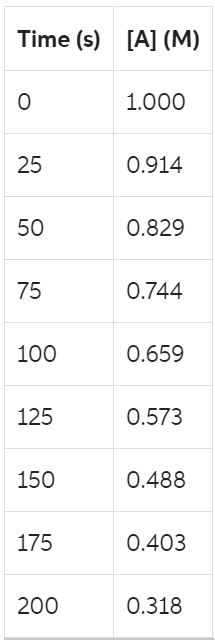# Problem: A reaction in which A → products was monitored as a function of time and the results are shown below.Determine the value of the rate constant.What is the rate of reaction when [A] = 0.20 M?

###### FREE Expert Solution

For the first part, we're being asked to determine the value of the rate constant.

For this, we will construct several graphs using the integrated rate law equations and select the graph that would generate a straight line

The integrated rate law for a zeroth-order reaction is as follows:

$\overline{){\mathbf{\left[}\mathbf{A}\mathbf{\right]}}_{{\mathbf{t}}}{\mathbf{=}}{\mathbf{-}}{\mathbf{kt}}{\mathbf{+}}{{\mathbf{\left[}}{\mathbf{A}}{\mathbf{\right]}}}_{{\mathbf{0}}}}$

y    =    mx   +  b

where:

[A]t = concentration at time t
k = rate constant
t = time
[A]0 = initial concentration

Graph time vs. [At]

90% (124 ratings)###### Problem Details

A reaction in which A → products was monitored as a function of time and the results are shown below.Determine the value of the rate constant.

What is the rate of reaction when [A] = 0.20 M?

Frequently Asked Questions

What scientific concept do you need to know in order to solve this problem?

Our tutors have indicated that to solve this problem you will need to apply the Rate Law concept. You can view video lessons to learn Rate Law. Or if you need more Rate Law practice, you can also practice Rate Law practice problems.